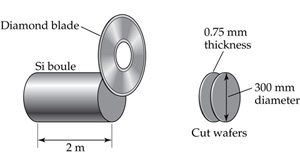# Problem: Silicon for computer chips is grown in large cylinders called “boules” that are 300 mm in diameter and 2 m in length, as shown. The density of silicon is 2.33 g/cm3. Silicon wafers for making integrated circuits are sliced from a 2.0 m boule and are typically 0.75 mm thick and 300 mm in diameter.What is the mass of a silicon wafer? (The volume of a cylinder is given by πr2h, where r is the radius and h is its height.)

###### FREE Expert Solution

Calculate the volume of a silicon wafer:

$\overline{)\mathbf{V}\mathbf{=}{\mathbf{\pi r}}^{\mathbf{2}}\mathbf{h}}$

h = 0.075 cm

96% (467 ratings)###### Problem Details
Silicon for computer chips is grown in large cylinders called “boules” that are 300 mm in diameter and 2 m in length, as shown.The density of silicon is 2.33 g/cm3. Silicon wafers for making integrated circuits are sliced from a 2.0 m boule and are typically 0.75 mm thick and 300 mm in diameter.

What is the mass of a silicon wafer? (The volume of a cylinder is given by πr2h, where r is the radius and h is its height.)

Frequently Asked Questions

What scientific concept do you need to know in order to solve this problem?

Our tutors have indicated that to solve this problem you will need to apply the Density of Geometric Objects concept. You can view video lessons to learn Density of Geometric Objects. Or if you need more Density of Geometric Objects practice, you can also practice Density of Geometric Objects practice problems.